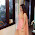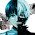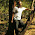## Thursday, 1 November 2012

### CBSE Class 7 - Maths - CH4 - Simple Equations (Ex 4.4)

Simple Equations

(NCERT Exercise 4.4)

Important Points:
An equation which has only one variable whose power is one is called a SIMPLE EQUATION.

In a simple equation keeping only the variable in the LHS of the equation and moving the constant to RHS (right hand side) is called the method of TRANSPOSITION.

Rules for method of Transposition are:
1. A positive constant in the LHS becomes a negative constant when it is taken to the RHS and vice versa.
2. A negative constant in the LHS becomes a positive constant when it is taken to the RHS and vice versa.
3. The coefficient of the variable in tbe LHS becomes t.he multiplicative inverse when it is taken to the RHS :and vice versa.
An equation remains the same if the LHS and the RHS are interchanged.

Q1:Set up equations and solve them to find the unknown numbers in the following cases
(a) Add 4 to eight times a number; you get 60.

Answer: Let number be = y
Eight times a number = 8y
Add 4 to to above result = 8y + 4
Required equation is: 8y + 4 = 60
⇒ 8y = 60 - 4                                    (Transposing 4 to RHS)
⇒ 8y = 56
⇒ y = 56/8
y = 7

Verification: LHS = 8y + 4 = 8 × 7 + 4 = 56 + 4 = 60 = RHS

(b) One fifth of a number minus 4 gives 3.

Answer: Let the number be = p
One fifth of number = p/5
∴ Required equation is:   p/5 - 4 = 3
Multiply by 5 to both sides,
⇒ (p/5 - 4) × 5 = 3 × 5
⇒  5 × p/5 - 4 × 5  = 15
⇒ p - 20 = 15
⇒ p = 15 + 20                       (transposing -20 to RHS)
p = 35

(c) If I take three fourths of a number and count up 3 more, I get 21.

Answer:Let the number be = t
Three fourth of number t = 3t/4
∴ Required equation is:  3t/4 + 3 = 21
Multiply by 4 to both sides
⇒ 4  × (3t/4 + 3 ) = 21 × 4
4 × 3t/4 + 4 × 3 =  84
⇒ 3t + 12 = 84
Transpose 12 to RHS
⇒ 3t = 84 - 12 = 72
⇒ t = 72/3
t = 24

(d) When I subtracted 11 from twice a number, the result was 15.

Answer: Let a number be y.
The desired equation is:  2y - 11 = 15
⇒ 2y = 15 + 11                        (Transposing -11 to RHS)
⇒ 2y = 26
⇒ y = 26/2
y = 13

(e) Munna subtracts thrice the number of notebooks he has from 50, he finds the result to be 8.

Answer:Let the number be = z
Thrice the number = 3z
Desired equation is:   50 - 3z  = 8
Transposing 50 to RHS
⇒ -3z = 8 - 50
⇒ -3z = -42
⇒ 3z = 42
⇒ z = 42/3
z = 14

(f) Ibenhal thinks of a number. If she adds 19 to it and divides the sum by 5, she will get 8.

Answer: Let the number is = m
Desired equation is:  (m + 19)/5 = 8
Multiply by 5 to both sides
⇒ 5 × (m+19)/5 = 5 × 8
⇒ m + 19 = 40
⇒ m = 40 - 19                (Transposing 19 to RHS)
m = 21

(g) Anwar thinks of a number. If he takes away 7 from 5/2 of the number, the result is 11/2 .

Answer:  Let the number be  = t
5/2 of the number = 5t/2
The desired equation is:

Q2: Solve the following:
(a) The teacher tells the class that the highest marks obtained by a student in her class is twice the lowest marks plus 7. The highest score is 87. What is the lowest score?

Answer:  Let the lowest score = y
Required equation is:
2 × lowest marks + 7 = 87
⇒ 2 × y + 7 = 87
⇒ 2y = 87 -7                (transposing 7 to RHS)
⇒ 2y = 80
Dividing both sides by 2,
⇒ 2y/2 = 80/2
∴ y = 40
Thus the lowest score is 40.

(b) In an isosceles triangle, the base angles are equal. The vertex angle is 40°. What are the base angles of the triangle? (Remember, the sum of three angles of a triangle is 180°).

Answer: Let the base angle = b°
Since, the sum of three angles of a triangle is 180°
Required equation:
2 × base angles + 40° = 180°
⇒ 2b + 40° = 180°
Transposing 40° to LHS
⇒ 2b = 180° - 40°
⇒ 2b =  140°
Dividing both sides by 2,
⇒   2b/2 = 140°/2
⇒ b = 70°
This the base angle is 70°

(c) Smita’s mother is 34 years old. Two years from now mother’s age will be 4 times Smita’s present age. What is Smita’s present age?

Answer: Let Smita's present age = m years
Present age of Smita's mother  = 34 years
After 2 yeats, Smita's mother will be = 34+ 2 = 36 years
Required equation is
4  × Smita's Present age = 36 years
4 × m  = 36
⇒ 4m  = 36
Dividing by 4 to both sides
⇒ 4m/4 = 36/4
⇒ m = 9
Thus Smita's present age = 9

(d) Sachin scored twice as many runs as Rahul. Together, their runs fell two short of a double century. How many runs did each one score?

Answer: Rahul scored the less number of runs.
Let Rahul's score = r runs
Sachin's score = 2 times of Rahul score = 2×r = 2r

Required equation is:
Rahul score + Sachin score + 2 = Double Century
⇒    r + 2r + 2 = 200
⇒    3r + 2 = 200
Transposing 2 to RHS
⇒    3r = 200 - 2
⇒    3r = 198
Divide both sides by 3
⇒    3r/3 = 198/3
⇒    r = 66
Thus Rahul score = 66                                  ... (answer)
and Sachin score = 2r = 2× 66 = 132            ... (answer)

Q3: Solve the following
(i) Irfan says that he has 7 marbles more than five times the marbles Parmit has. Irfan has 37 marbles. How many marbles does Parmit have?

Answer: Let the number of marbles Parmit has = n
Number of marbles Irfan has = 37
Required Equation is:
5 times of Parmit Marbles + 7 = Irfan's Marbles
⇒ 5 × n + 7 = 37
⇒ 5n + 7 = 37
Transposing 7 to RHS
⇒ 5n = 37 - 7
⇒ 5n = 30
Dividing by 5 to both sides
⇒ 5n/5 = 30/5
⇒ n = 6
Thus Parmit has 6 marbles.

(ii) Laxmi's father is 49 years old. He is 4 years older than three times Laxmi's age. What is Laxmi's age?

Answer:  Let Laxmi's age = m years
Age of Laxmi's father = 49
Required Equation is:
Three times Laxmi Age + 4 = Laxmi's Father Age
⇒ 3 × m + 4 = 49
3m + 4 = 49
Transposing 4 to RHS

3m = 49 - 4

⇒ 3m = 45
Divide by 3 to both sides,
⇒ 3m/3 = 45/3
m = 15

Thus Laxmi's age = 15 years.

(iii) Maya, Madhura and Mohsina are friends studying in the same class. In a class test in geography, Maya got 16 out of 25. Madhura got 20. Their average score was 19. How much did Mohsina score?

Answer: Let Mohsina's score = t
Total score = 25
Maya score = 16
Madhura score = 20
Average score = 19
Required equation is:

(Maya Score + Madhura score + Mohsina Score) ÷ 3 = Average score
⇒ (16 + 20 + t )/3 = 19
Multiple both sides by 3,
⇒  3 × (16 + 20 + t )/3 = 3 × 19
⇒ 16 + 20 + t = 57
⇒ t + 36 = 57
Transposing  36 to RHS
⇒ t = 57 - 36
⇒ t = 21
Thus, Mohsina scored 21 marks.

(iv) People of Sundargram planted a total of 102 trees in the village garden. Some of the trees were fruit trees. The number of non-fruit trees were two more than three times the number of fruit trees. What was the number of fruit trees planted?

Answer: Let number of fruit trees = u.
Total number of trees = 102
Non-fruit trees = 3 × fruit trees + 2 = 3u + 2
Required equation is:
Fruit Trees + Non-Fruit Trees = 102
⇒ u + (3u + 2) = 102
⇒ u + 3u + 2 = 102
⇒ 4u + 2 = 102
Transposing 2 to RHS
4u = 102 -2
4u = 100
Divide by 4 to both sides
4u/4 = 100/4
⇒ u = 25
Thus number of fruit trees planted = 251.eeeeeeeeeesssssyyyyyyyy peesssssssssyyyyyyy!!!!!!
gosh!! i could solve all your questions is 1.26 minuets by the timer......... something tough would attract loads and loads of people to try this out and not only that you should give some space for people to type the answers here and you should use a timer and also check the correct and wrong answers and give marks and medals accordingly on this site...... make this an ipad ipod iphone game so people could actually connect this to fb and express their intelligence in front of their fb friends and also make this paper a lot more tough next time when i come here i want to take 10 minuets for the same number of questions. go on..!!!!! make them tough....... you'll earn a lot by all that k!! now i stop my ttips. wanna follow them then do it. they are actually going to help you im 100% sure!!!!!!! i took 1.13 minuets to type this full thing on my laptop!!! hahahaha!!!! :-)

1.learn the spelling of "minutes" before bluffing

2.coooooooooooolllll naaaaaaa!!!! kkkk! mail me nyway wen u change the proceedings!!! now i took 3.12 seconds to type this !!!!!! anyone faster than me???????????? :-P

1.2.typed "this" in 0. 3 seconds

3.wow!!!! I never knew that the questions from my worksheets could be found in this blog! this is amazingly amazing!!!

4.5.checkout this blog.. its for helping students....http://extraquestions.blogspot.in/

6.7.8.Good way of solving problems, helpful for students.

9.10.This comment has been removed by the author.

11.12.awesome,now my exams are toooooo.... easy for me to solve.(YES)
coooooooooooooooollllllll

13.answered in 1 min 2 seconds

14.hi thareek sir
danish.n.shariff

15.